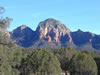# Captions

## text wraps around imageCaption

This is the static Content : This is the static Content : This is the static Content : This is the static Content : This is the static Content : This is the static Content : This is the static Content : This is the static Content :Caption

This is the static Content : This is the static Content : This is the static Content : This is the static Content : This is the static Content : This is the static Content : This is the static Content : This is the static Content :Caption

This is the static Content : This is the static Content : This is the static Content : This is the static Content : This is the static Content : This is the static Content : This is the static Content : This is the static Content :Caption

This is the static Content : This is the static Content : This is the static Content : This is the static Content : This is the static Content : This is the static Content : This is the static Content : This is the static Content :Caption

This is the static Content : This is the static Content : This is the static Content : This is the static Content : This is the static Content : This is the static Content : This is the static Content : This is the static Content :